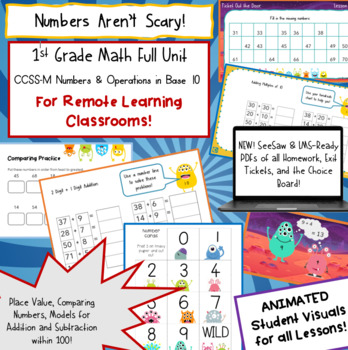# 1st Grade Place Value--REMOTE edition1st - 2nd
Subjects
Standards
Resource Type
Formats Included
•Google Drive™ folder### Description

NEW to this Unit! If you loved our beginning of the year review, this unit provides the next set of lessons, AND we have also included PDFs of all homework, exit tickets, and the choice board in a format that is SeeSaw-friendly!

This two+ week, monster-themed lesson plan pack comes with printables, online activities, and digital versions of practice that will help your first graders tackle place value and addition. It also includes all your lessons in a ready-to-go animated Google Slideshow, so you can demonstrate games and strategies in a remote classroom environment.

This unit was designed to cover all the first grade standards for Numbers and Operations in Base Ten:

Extend the counting sequence.

Count to 120, starting at any number less than 120. In this range, read and write numerals and represent a number of objects with a written numeral.

Understand place value.

Understand that the two digits of a two-digit number represent amounts of tens and ones. Understand the following as special cases:

• 10 can be thought of as a bundle of ten ones — called a "ten."
• The numbers from 11 to 19 are composed of a ten and one, two, three, four, five, six, seven, eight, or nine ones.
• The numbers 10, 20, 30, 40, 50, 60, 70, 80, 90 refer to one, two, three, four, five, six, seven, eight, or nine tens (and 0 ones).

Compare two two-digit numbers based on meanings of the tens and ones digits, recording the results of comparisons with the symbols >, =, and <.

Use place value understanding and properties of operations to add and subtract.

Add within 100, including adding a two-digit number and a one-digit number, and adding a two-digit number and a multiple of 10, using concrete models or drawings and strategies based on place value. Understand that in adding two-digit numbers, one adds tens and tens, ones and ones; and sometimes it is necessary to compose a ten.

Given a two-digit number, mentally find 10 more or 10 less than the number, without having to count; explain the reasoning used.

Subtract multiples of 10 in the range 10-90 from multiples of 10 in the range 10-90 (positive or zero differences)

Total Pages
Included
Teaching Duration
2 Weeks
Report this Resource to TpT
Reported resources will be reviewed by our team. Report this resource to let us know if this resource violates TpT’s content guidelines.

### Standards

to see state-specific standards (only available in the US).
Count to 120, starting at any number less than 120. In this range, read and write numerals and represent a number of objects with a written numeral.
Understand that the two digits of a two-digit number represent amounts of tens and ones. Understand the following as special cases:
10 can be thought of as a bundle of ten ones - called a “ten.”
The numbers from 11 to 19 are composed of a ten and one, two, three, four, five, six, seven, eight, or nine ones.
The numbers 10, 20, 30, 40, 50, 60, 70, 80, 90 refer to one, two, three, four, five, six, seven, eight, or nine tens (and 0 ones).